Next: Electromagnetic momentum Up: Electromagnetic energy and momentum Previous: Introduction

## Energy conservation

We have seen that the energy density of an electric field is given by [see Eq. (595)]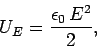(1018)

whereas the energy density of a magnetic field satisfies [see Eq. (950)]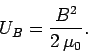(1019)

This suggests that the energy density of a general electromagnetic field is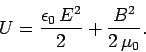(1020)

We are now in a position to demonstrate that the classical theory of electromagnetism conserves energy. We have already come across one conservation law in electromagnetism: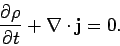(1021)

This is the equation of charge conservation. Integrating over some volume, bounded by a surface, and making use of Gauss' theorem, we obtain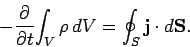(1022)

In other words, the rate of decrease of the charge contained in volumeequals the net flux of charge across surface. This suggests that an energy conservation law for electromagnetism should have the form(1023)

Here,is the energy density of the electromagnetic field, and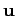is the flux of electromagnetic energy (i.e., energyper unit time, per unit cross-sectional area, passes a given point in the direction of). According to the above equation, the rate of decrease of the electromagnetic energy in volumeequals the net flux of electromagnetic energy across surface.

However, Eq. (1023) is incomplete, because electromagnetic fields can gain or lose energy by interacting with matter. We need to factor this into our analysis. We saw earlier (see Sect. 5.3) that the rate of heat dissipation per unit volume in a conductor (the so-called ohmic heating rate) is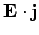. This energy is extracted from electromagnetic fields, so the rate of energy loss of the fields in volumedue to interaction with matter is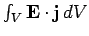. Thus, Eq. (1023) generalizes to(1024)

From Gauss' theorem, the above equation is equivalent to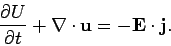(1025)

Let us now see if we can derive an expression of this form from Maxwell's equations.

We start from the differential form of Ampère's law (including the displacement current):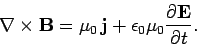(1026)

Dotting this equation with the electric field yields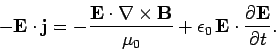(1027)

This can be rewritten(1028)

Now, from vector field theory(1029)

so(1030)

The differential form of Faraday's law yields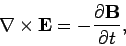(1031)

so(1032)

This can be rewritten(1033)

Thus, we obtain the desired conservation law,(1034)

where(1035)

is the electromagnetic energy density, and(1036)

is the electromagnetic energy flux. The latter quantity is usually called the Poynting flux, after its discoverer.

Let us see whether our expression for the electromagnetic energy flux makes sense. We all know that if we stand in the sun we get hot (especially in Texas!). This occurs because we absorb electromagnetic radiation emitted by the Sun. So, radiation must transport energy. The electric and magnetic fields in electromagnetic radiation are mutually perpendicular, and are also perpendicular to the direction of propagation(this is a unit vector). Furthermore,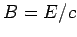. Equation (447) can easily be transformed into the following relation between the electric and magnetic fields of an electromagnetic wave: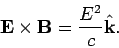(1037)

Thus, the Poynting flux for electromagnetic radiation is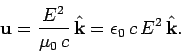(1038)

This expression tells us that electromagnetic waves transport energy along their direction of propagation, which seems to make sense.

The energy density of electromagnetic radiation is(1039)

using. Note that the electric and magnetic fields have equal energy densities. Since electromagnetic waves travel at the speed of light, we would expect the energy flux through one square meter in one second to equal the energy contained in a volume of length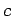and unit cross-sectional area: i.e.,times the energy density. Thus,(1040)

which is in accordance with Eq. (1038).

In the presence of diamagnetic and magnetic media, starting from Eq. (860), we can derive an energy conservation law of the form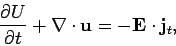(1041)

via analogous steps to those used to derive Eq. (1034). Here, the electromagnetic energy density is written(1042)

which is consistent with Eq. (1035). The Poynting flux takes the form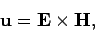(1043)

which is consistent with Eq. (1036). Of course, the above expressions are only valid for linear dielectric and magnetic media.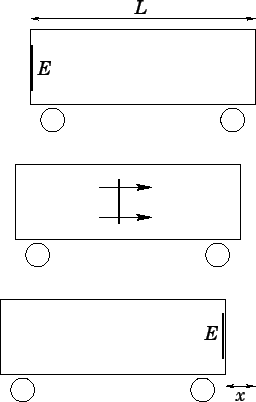Next: Electromagnetic momentum Up: Electromagnetic energy and momentum Previous: Introduction
Richard Fitzpatrick 2006-02-02